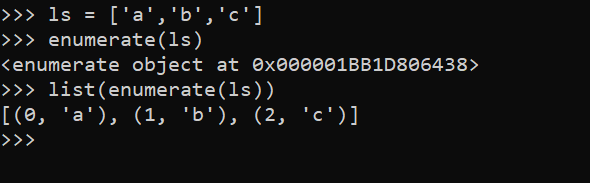# Python中的枚舉函數enumerate()的具體用法

• enumerate()是python的內置函數、適用於python2.x和python3.x
• enumerate在字典上是枚舉、列舉的意思
• enumerate參數為可遍歷/可迭代的對象(如列表、字符串)
• enumerate多用於在for循環中得到計數，利用它可以同時獲得索引和值，即需要index和value值的時候可以使用enumerate
• enumerate()返回的是一個enumerate對象

python中最常用的數據結構就是list，處理list中每個元素，通常都用for循環搞定。```ls = ['a', 'b', 'c']
# method 1
for i in range(len(ls)):
print(i， end=' ')
print(ls[i])

# method 2
for s in ls:
print(ls.index(s), end=' ')
print(s)

# method 3
for i, s in enumerate(ls):
print(i, end=' ')
print(s)
```

### enumerate的使用：

0,1
1,2
2,3
3,4
4,5
5,6

```>>> lst = [1,2,3,4,5,6]
>>> for index,value in enumerate(lst):
print ('%s,%s' % (index,value))

0,1
1,2
2,3
3,4
4,5
5,6```
```#指定索引從1開始
>>> lst = [1,2,3,4,5,6]
>>> for index,value in enumerate(lst,1):
print ('%s,%s' % (index,value))

1,1
2,2
3,3
4,4
5,5
6,6

#指定索引從3開始
>>> for index,value in enumerate(lst,3):
print ('%s,%s' % (index,value))

3,1
4,2
5,3
6,4
7,5
8,6```

### 補充：

```count = len(open(filepath, 'r').readlines())
```

```count = 0
for index, line in enumerate(open(filepath,'r'))：
count += 1```